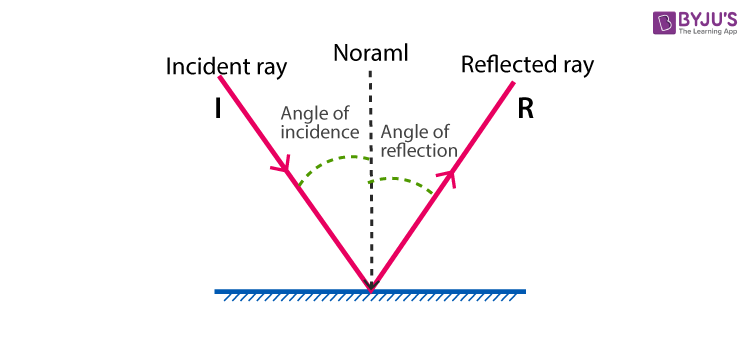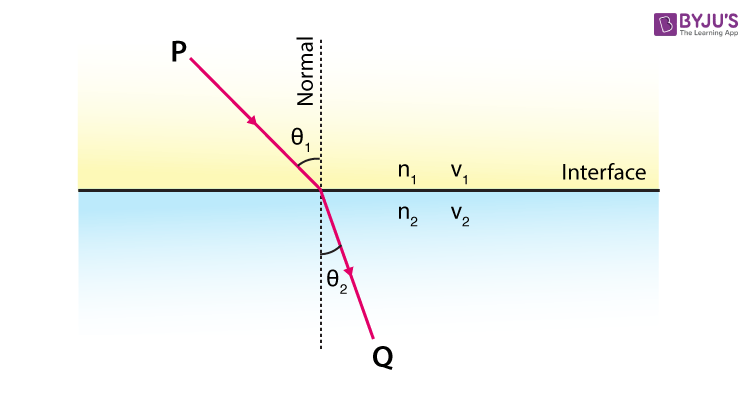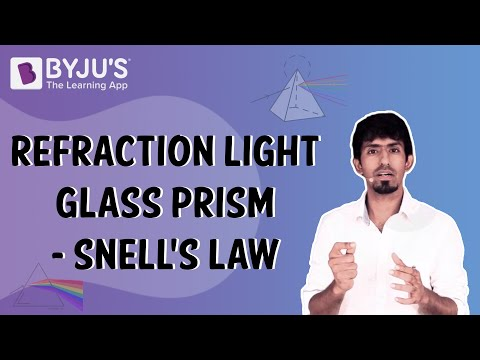Checkout JEE MAINS 2022 Question Paper Analysis : Checkout JEE MAINS 2022 Question Paper Analysis :

# Geometric Optics

## What is Geometric Optics?

When a ray of light is reflected by some angle by a barrier in its pathway, it is said that the light beam is rebounded and this procedure is known as a reflection of light.

When the beam of light crosses from one medium to the other, it diverges slightly from its route. This deviation hinges over the medium where the light is crossing through. This procedure is termed as the refraction of light.

### Snell’s Law (Laws of Refraction and Reflection):

When light is refracted or reflected, one can check the basis of procedure for refraction and reflection along with the things linked to them.

### The Laws of Reflection in Geometric Optics:

The conduct of the ray of light that is rebounded by a smooth mirror is judged based on the laws of reflection. But primarily, one needs to know the basic terms.

Light approaching a mirror is termed an incident ray and the beam of light that is reflected by the mirror is termed as a reflected ray. At the spot of reflection, the perpendicular that is drawn is termed as normal. The angle amidst the incident ray and normal is the angle of incidence, while the angle amidst the reflected ray and normal is the angle of reflection.

Conferring to the laws of reflection, the angle of incidence is always equivalent to the angle of reflection.The law of refraction is also termed as Snell’s Law. Conferring to this law, “the ratio between the sine of the angle of incidence and sine of the angle of refraction in two separate mediums wherein the light travels equal to the ratio of the mediums phase velocities.” It is also equivalent to the reciprocal ratio between the indices of refraction.i.e,

$$\begin{array}{l}\frac{sin\; \Theta _{1}}{sin\; \Theta _{2}}=\frac{v_{1}}{v_{2}}=\frac{\lambda _{1}}{\lambda _{2}}=\frac{n_{1}}{n_{2}}\end{array}$$### Refractive Index of the Medium in Geometric Optics:

The refractive index is denoted by ‘n’ and described as a dimensionless number which shows that the light or any radiation travels through a medium. It is mathematically expressed as:

n = c/v

Where,

‘v’ indicates the phase velocity of light in a medium, and

‘c’ indicates the speed of light in a vacuum.

### Refraction through a Glass Prism:

The ray of light comprises different colors and also called white light. When the white light crosses through a prism, it is disintegrated into the colors it comprises, which is violet, indigo, blue, green, yellow, orange, and red. This procedure is also termed as dispersion that occurs sue to a different wavelength of each color. Because of these discrete wavelengths, the dispersed ray from the prism refracts into diverse angles and therefore displaying all the colors that are present in the white light. This color configuration is also termed as VIBGYOR titled with the first letter in the order of diminishing deviations.